# Engineering Mechanics - KOP: Force and Acceleration

### Exercise :: KOP: Force and Acceleration - General Questions

• KOP: Force and Acceleration - General Questions
6.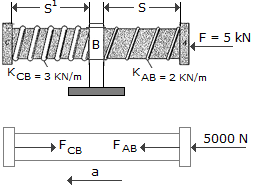The 2-kg shaft CA passes through a smooth journal bearing at B. Initially, the springs, which are coiled loosely around the shaft, are unstretched when no force is applied to the shaft. In this position s = sª = 250 and the shaft is originally at rest. If a horizontal force of F = 5 kN is applied, determine the speed of the shaft at the instant s = 50 mm, sª = 450 mm. The ends of the springs are attached to the bearing at B and the caps at C and A.

 A. v = 31.6 m/s B. v = 14.14 m/s C. v = 44.7 m/s D. v = 30.0 m/s

Explanation:

No answer description available for this question. Let us discuss.

7.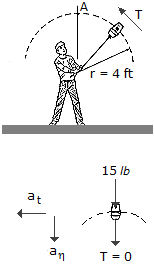A boy twirls a 15-lb bucket of water in a vertical circle. If the radius of curvature of the path is 4 ft, determine the minimum speed the bucket must have when it is overhead at A so no water spills out.

 A. v = 11.35 ft/s B. v = 0 C. v = 6.26 ft/s D. v = 2.83 ft/s

Explanation:

No answer description available for this question. Let us discuss.

8.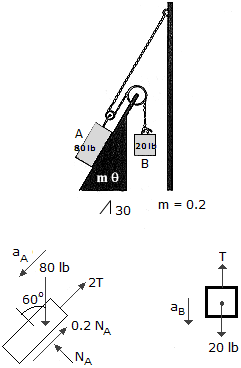Determine the acceleration of block A when the system is released. The coefficient of friction and the weight of each block are indicated in the figure. Neglect the mass of the pulleys and cords.

 A. aA = 7.50 ft/s2 Up the slope B. aA = 7.50 ft/s2 Down the slope C. aA = 4.28 ft/s2 Up the slope D. aA = 4.28 ft/s2 Down the slope

Explanation:

No answer description available for this question. Let us discuss.

9.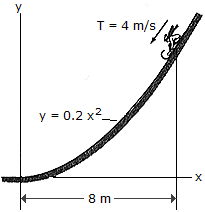A tobbogan and rider have a total mass of 100 kg and travel down along the (smooth) slope defined by the equation y = 0.2x2. At the instant x = 8 m, the toboggan's speed is 4 m/s. At this point, determine the rate of increase in speed and the normal force which the toboggan exerts on the slope. Neglect the size of the toboggan and rider for the calculation.

 A. at = 8.32 m/s2, N = 520 N B. at = 8.32 m/s2, N = 537 N C. at = 9.36 m/s2, N = 310 N D. at = 9.36 m/s2, N = 293 N

Explanation:

No answer description available for this question. Let us discuss.

10.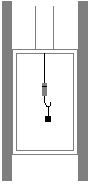A block having a mass of 2 kg is placed on a a spring scale located in an elevator that is moving downward. If the scale reading, which measures the force in the spring, is 20 N, determine the acceleration of the elevator. Neglect the mass of the scale.

 A. a = 10.00 m/s2B. a = 0.1900 m/s2C. a = 0.1900 m/s2D. a = 10.00 m/s2